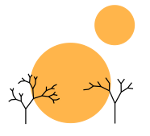# Rigid Coxeter Groups

Rimu Shuang. "Untitled Image". Dec 3, 2012. Under a Creative Commons Attribution 3.0 License.

### math

The study of the rigidity of Coxeter groups is motivated by the observation that for Coxeter systems (W, S), isomorphisms of the underlying Coxeter groups do not guarantee isomorphisms of the Coxeter systems themselves. This is most easily seen in the diagrams associated with Coxeter systems; the same Coxeter group with two different sets of Coxeter generators can give rise to dramatically different Coxeter diagrams. The natural question then arises in which cases does the group structure of a Coxeter system in fact determine the associated Coxeter diagram? From this question comes the natural definitions rigid Coxeter groups.

Definition: A rigid Coxeter group is a Coxeter group W with simple roots S such that the Coxeter System (W, S) gives rise to the same Coxeter diagram (up to isomorphism) regardless of the S chosen. Equivalently a rigid Coxeter group always has an automorphism on W which takes any choice of Coxeter generators S to any other choice of Coxeter generators S (assuming S and S both give rise to the same group).

There is a stronger condition for Coxeter groups than rigidity known as strong rigidity. A strongly rigid Coxeter group is a rigid Coxeter group in which given any choice of Coxeter generators S and S, there exists an inner automorphism on the group taking S to S.
\end{definition}

In order to show that these definitions are meaningful, it is instructive to consider both examples of and non-examples of rigid Coxeter groups and strongly rigid Coxeter group. The Cartesian product of the group of two elements, i.e. (C2)n is a rigid Coxeter group. To see this, identify C2 with the field of two elements 𝔽2. Then we can create the vector space (𝔽2)n over 𝔽2. We can embed (𝔽2)n in n and from that we can use (C2)n’s natural group action on n of the following: Given (c1, …cn) ∈ (C2)n and (r1, …, rn) ∈ ℝn and (c1, …, cn)(r1, …, rn) = (r1′, …, rn′), if ci = 0 then ri′ = ri otherwise if ci = 1 then ri′ =  − ri. We note that our simple reflections S are naturally identified with the simple roots which in turn are a basis for (𝔽2)n (via linear independence). Since our only choice of scalars is 1 or 0, the vector space automorphisms of (𝔽2)n are precisely the group automorphisms of (C2)n. Then because for any two bases there always exists a vector space automorphism mapping one to the other, for any two Coxeter generator sets S and S, there must always be a group automorphism mapping one to the other.

Note, however, that (C2)n is not strongly rigid. Its abelian nature means that the only inner automorphism is the identity map.

An example of a strongly rigid Coxeter group is D3, i.e. the symmetries of the triangle. Note that any Coxeter generator must be a reflection, not a rotation (since there are no 180 rotations possible on a triangle). Note too that two reflections are necessary to generate the Coxeter group and that each plane fixed by the reflection is separated by 120 from the plane fixed by the other reflection. Then it becomes geometrically apparent that conjugating by a rotation r is sufficient to take any pair of reflections to another pair of reflections.

More about rigid Coxeter groups will be posted soon.